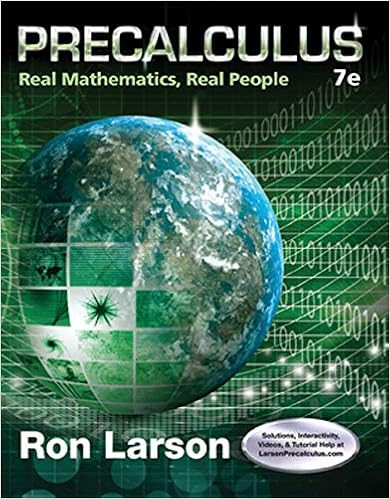# B 10 pts what is the final angular velocity magnitude

• Test Prep
• 9
• 100% (3) 3 out of 3 people found this document helpful

This preview shows page 8 - 9 out of 9 pages.

##### We have textbook solutions for you!
The document you are viewing contains questions related to this textbook.The document you are viewing contains questions related to this textbook.
Chapter 6 / Exercise 6
Precalculus: Real Mathematics, Real People
LarsonExpert Verified
b)(10 pts.)What is the final angular velocity (magnitude and direction) of the rod?
'
c)(10 pts.) What is the change in kinetic energy of the system (the rod plus particle)?
##### We have textbook solutions for you!
The document you are viewing contains questions related to this textbook.The document you are viewing contains questions related to this textbook.
Chapter 6 / Exercise 6
Precalculus: Real Mathematics, Real People
LarsonExpert Verified
Problem 5 (27 pts.): A block of mass m1rests on a frictionless inclined plane at a height above a flat horizontal plane. This mass is released and slides down the incline, eventually colliding elastically with a second block at rest at x 0 with mass m2, with m1 = 3m2/4. Immediately after the elastic collision, block number 2 moves to the right with velocity v2f. It encounters a region that has friction with a coefficient of kinetic friction ofμk, traveling a distancedbefore coming to rest. Answer the following questions about these two blocks. a)(7 pts.)Determine an equation for v2fimmediately after the collision (using m2, h, and h = g)
2
b)(7 pts.)Determine an equation for d(in terms of m2, h,μk, and g), the distance that block 2
c)(6 pts.)What is the magnitude (equation) and sign of the work done by the frictional force on block 2?
d)(7 pts.) What is the velocityvfof block 1 after the collision (in terms of m2, h, and g). Use vector notation. 1
1
•••